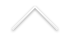Partial Differential Equations - Research in the field of partial differential equations focuses on the mathematical investigation of the solutions of equations or systems of differential equations and their properties. The problems addressed include existence, uniqueness, stability, regularity, asymptotic behavior and convergence of approximation schemes for the solutions. The techniques used come primarily from mathematical analysis, such as functional analysis, measure theory and harmonic analysis. It is a field where mathematics has a natural interface with many areas of application, including Physics, Chemistry, Biology, Economy and Finance, Engineering and even Social Sciences. Many of the fundamental laws and models in science and technology are naturally expressed as systems of partial differential equations.

Integrantes do grupo de pesquisa:

• Milton Lopes
• Helena Nussenzveig Lopes
• Flavio Dickstein
• Fabio Ramos
• Ricardo Rosa
• Xavier Carvajal
• Pedro Gamboa